Teacher: Richard
Customers Who Have Viewed This Course: 2145
\$179.99

0 Welcome to Algebra for College-Bound 11th Graders 03:19

A brief introduction to this course.

-1 Basic Algebra Review 11:02

A list of critical basic algebra vocabulary and processes that need to be mastered before beginning this course.

1 Lesson 1 – Set Theory 05:13

Numbers that share specific characteristics can be "put" into unique sets. It's not unlike a set of dishes; each dish shares a certain characteristic. It's the same concept with numbers; a set of numbers are a group of numbers that share a common characteristic.2 Lesson 2 - Number Sets 12:35

The main Number Sets that we use today.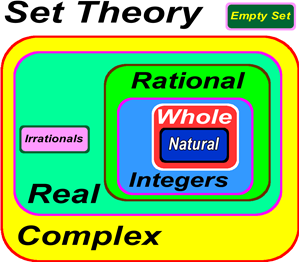4 Lesson 3 – Symbols Used in Set Theory 02:48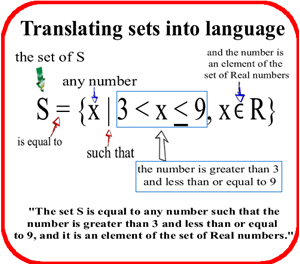5 Lesson 4 - Set Notation 12:35

Set Notation

There are many ways to represent a set of numbers. Below are the main two types of set notation.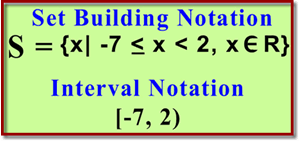7 Lesson 5 - Subsets 03:07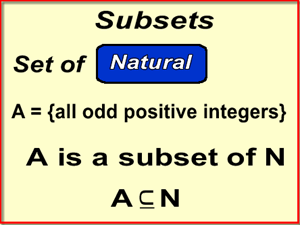Sets and Subsets

Any set "contained" inside another set is called a subset. Another way to think about a subset is any set which all the elements of a set are contained in another set.

Example:  Let A = {1, 3, 5, 7} and let B = {x|x ∈ N}, then A is a subset of B (all elements of A are contained in B).

We write subsets like this: A ⊆ B  (The set A is a subset of set B.)

A proper subset is a subset that does not contain every element of the original set. So, we can also say that the set A is a proper subset of set B: A ⊂ B.

Example: The set of Natural numbers is a proper subset of the set of Whole numbers because the set of Natural numbers does not contain the number 0.

N ⊂ W (The set of Natural numbers is a proper subset of the set of Whole numbers.)

All sets are also subsets of themselves, but not proper subsets of themselves.

Example: N ⊆ N (The set of Natural numbers is a subset of itself.)

8 Lesson 6 - Unions and Intersections of Sets 06:57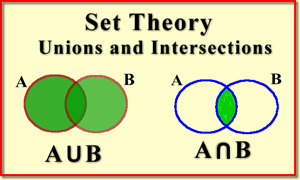10 Lesson 7 - Venn Diagrams 10:00

Venn Diagrams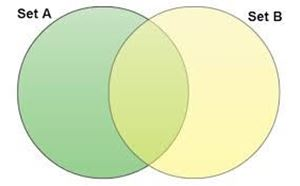12 Section 1: Set Theory Quiz 00:00

Section 1: Set Theory Quiz (Answers under Resources)

13 Section 1 - Brain Candy 02:50

Proof that you are what you are not!

14 Lesson 1 - The Cartesian Plane 03:41

The Cartesian Plane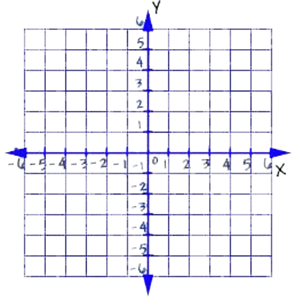15 Lesson 2 - The Fundamental Graphing Principle 02:16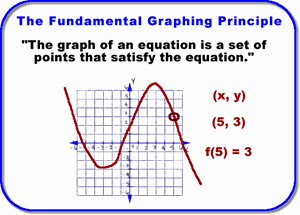16 Lesson 3 - Vertical and Horizontal Lines on a Graph 01:58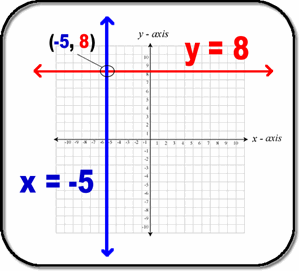18 Lesson 4 - Reflections on a Graph 04:36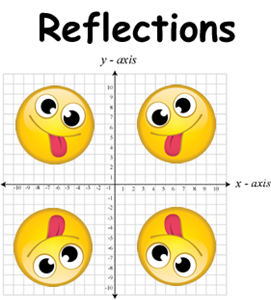20 Lesson 5 - Intercepts 04:24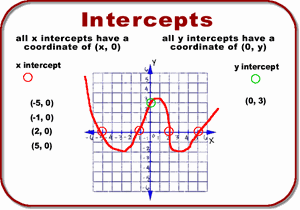Intercepts Worksheet Review Video ans answers

22 Lesson 6 - Midpoints 04:14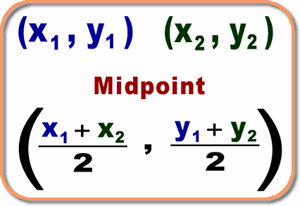23 Lesson 7 - Distance Formula 04:10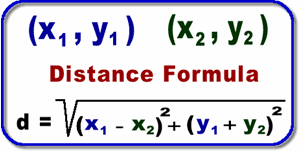25 Lesson 8 - Finding points on a graph with a fixed distance 04:34

Finding points on a graph with a fixed distance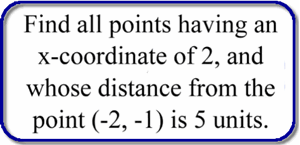26 Lesson 9 - Triangle XYZ is a right triangle. 03:41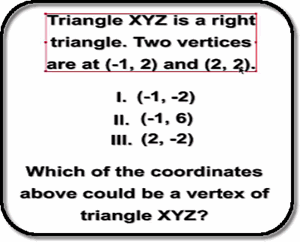27 Lesson 10 - Find the third vertex of a 30-60-90 degree triangle 04:34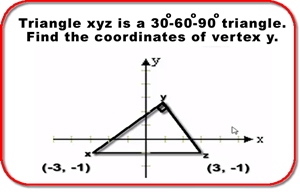28 Lesson 11 - Find the fourth point of a square 05:23

Find a point D(x; y) such that the points A(-3; 1), B(4; 0), C(0;-3) and D are the corners of a square.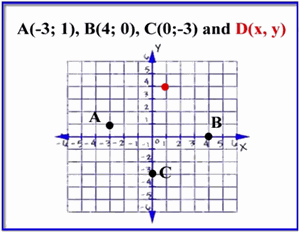29 Lesson 12 - Area of circle and a triangle 04:56

Circle C passes through the three vertices of triangle T. If the vertices of triangle T are (-1, 2), (1, 6), and (5, 4), then what is the area of cirlce C?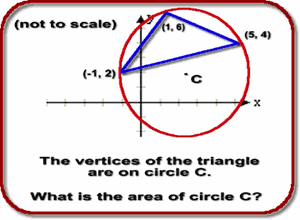30 Lesson 13 - Right triangle problem no coordinates 04:09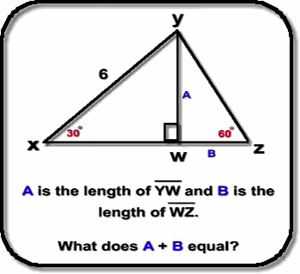31 Coordinate Geometry Worksheet 02:50

The Cartesian Plane and Coordinate Geometry Worksheet

33 Section 2: Cartesian Plane and Coordinate Geometry Quiz 02:03

Section 2: Cartesian Plane and Coordinate Geometry Quiz (Answers under Resources)

34 Section 2 - Brain Candy 01:50

How did the right triangle get its name?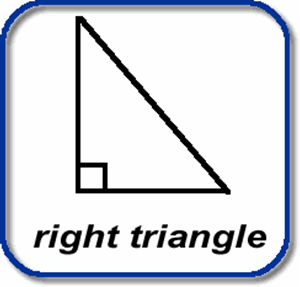35 PRACTICE Midterm 1 00:00

PRACTICE Midterm 1

38 Lesson 1 - Relations 05:4539 Lesson 2 - Relations Graphing using Set Notation 14:48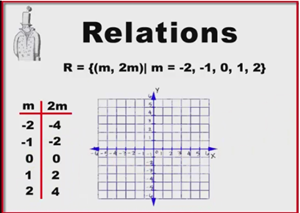40 Lesson 3 - Set notation in a plane 08:05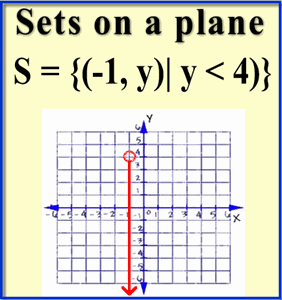42 Lesson 3 - Function notation 07:32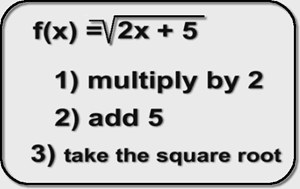43 Lesson 4 - Domains and Ranges of Functions 04:53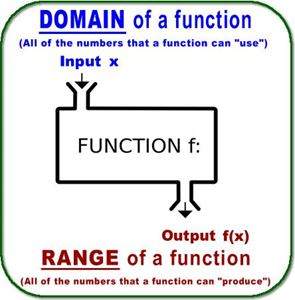45 Lesson 5 - Linear Functions: SLOPE 03:46

Linear Functions: SLOPE

46 Lesson 6 - Linear Functions GRAPHING 07:17

You remember the the slope-intercept equation for a straight line? It's our old friend ... y = mx + b

47 Lesson 7 - Linear Equations: SOLVING 04:28

How to algebraically write out a linear equation.

48 Lesson 8 - Linear inequalities: GRAPHING 02:54

How to graph linear inequalities.

50 Lesson 9 - Step-wise Functions (Piece-wise) 10:15

A step-wise function can be thought of as a "multiple" function; which function to use depends on the value of the number (x) being plugged into the step-wise function.

52 Lesson 11 - Absolute Value Functions 10:25

Absolute value functions are very common in mathematics. They can be tricky!

54 Lesson 11 - Absolute Value Inequalities 07:46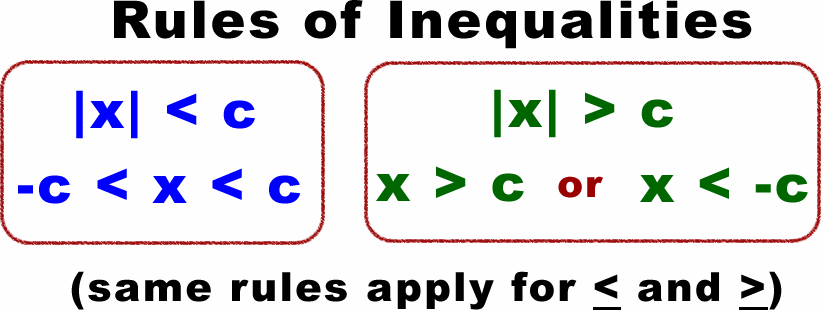55 Lesson 12 - Absolute Value Inequalities GRAPHING 03:25

How to graph an inequality equation.

57 Section 3: Functions (General) and Graphing Quiz 00:38

Section 3: Functions (General) and Graphing Quiz  (Answers under Resources)

58 Section 3 - Brain Candy 02:47

Section 3 - Brain Candy

59 Lesson 1 - Graphing Quadratic Functions 04:15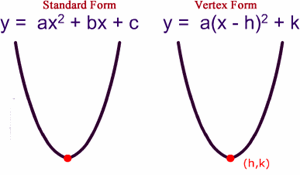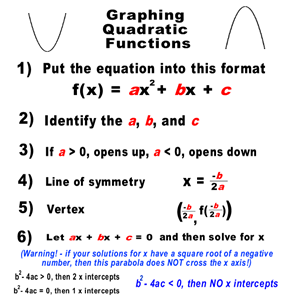61 Lesson 2 - Graphing Quadratics with x intercepts 04:02

Graphing Quadratic Functions with x intercepts

63 Lesson 3 - Imaginary Numbers 03:32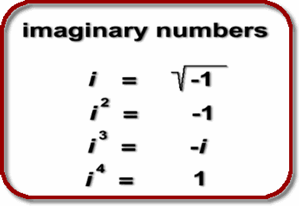64 Lesson 4 - Complex Numbers 04:44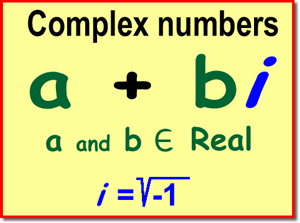66 Lesson 5 - Quadratics with No X Intercepts 07:52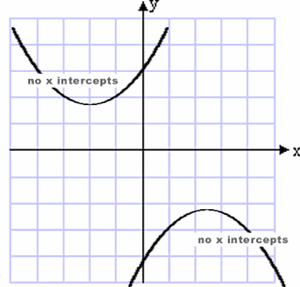68 Lesson 6 - Solving Quadratics by Completing the Square 05:28

Solving Quadratic Equations by Completing the Square

73 Lesson 9 - Minimums and maximums of quadratic functions 12:05

Minimums and maximums of quadratic functions.

74 Lesson 10 - Maximization of quadratics word problems 04:42

Farmer Skippy has 32 linear feet of fencing and he wants to fence in his yard so that it will maximize the area. He decides on a rectangular yard but he only needs three sides because the fourth side will be the wall of his house. Find the dimensions of the sides and the maximum area of the yard.

77 Section 4 - Brain Candy 03:14

Section 4 - Brain Candy

78 PRACTICE Midterm 2 00:00

PRACTICE Midterm 2

81 Lesson 1 - Fundamental Theorem of Algebra 07:12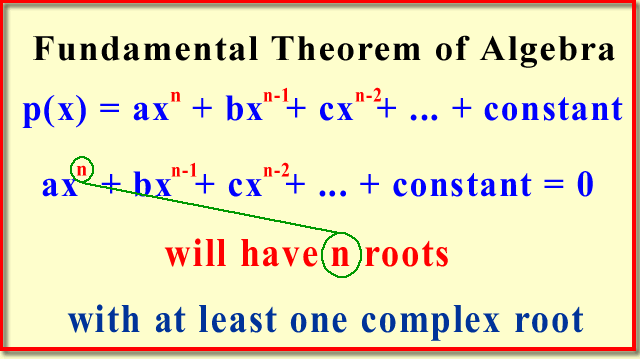82 Lesson 2 - Polynomial Function Definition 03:22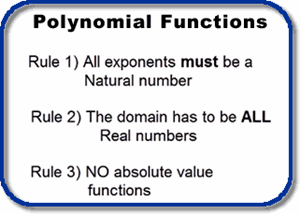83 Lesson 3 - What is a Polynomial Function? 04:44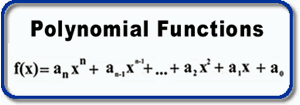85 Lesson 4 - Function Arithmetic 06:35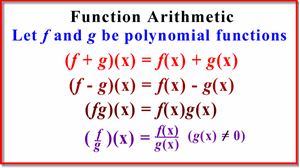87 Lesson 5 - Finding a “Zero” of a Function 04:45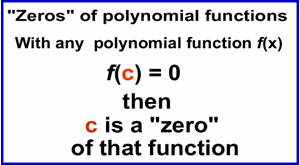89 Lesson 6 - Multiplicities of a Zero 01:54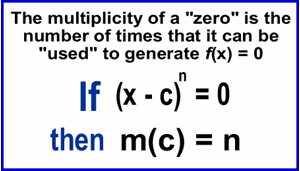91 Lesson 7 - The Factor and The Remainder Theorems 02:14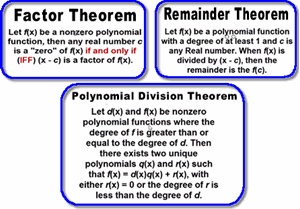92 Lesson 8 - Polynomial Division 05:02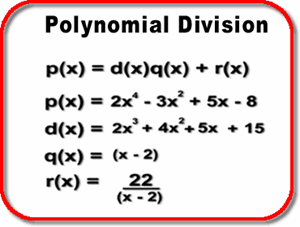94 Lesson 9 - Synthetic Polynomial Division 08:24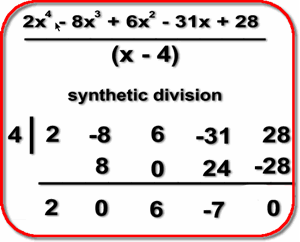95 Lesson 9b - Synthetic Polynomial Division example 04:3196 Synthetic Polynomial Division Worksheet 00:00

Synthetic Polynomial Division Worksheet

98 Lesson 10 - Difference Quotient Function 06:32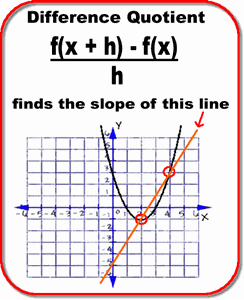100 Lesson 11 - Average Rate of Change Function 04:49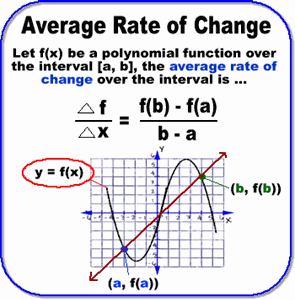102 Lesson 12 - Function Composition 04:38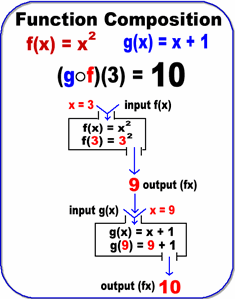104 Lesson 14 - One-to-one and Onto Functions 11:25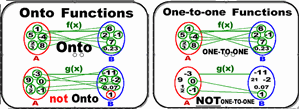106 Lesson 15 - Inverse Functions 05:13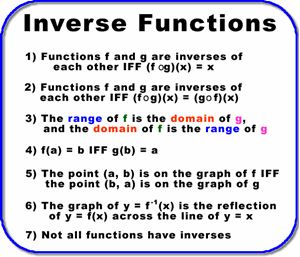108 Section 5: Polynomial Functions Quiz 01:19

Section 5: Polynomial Functions Quiz (Answers under Resources)

109 Section 5 - Brain Candy 04:01

Section 5 - Brain Candy

110 Lesson 1 - Rules of Exponents (Review) 02:06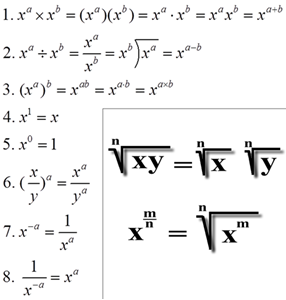111 Lesson 2 - Rational Exponents 08:36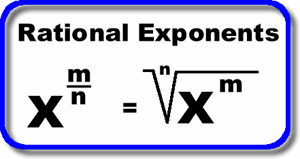113 Lesson 3 - Exponential Equations 02:56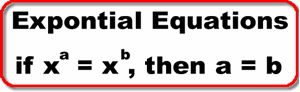114 Powers of Numbers 00:00

Powers of Numbers

116 Lesson 4 - Properties of Exponential Functions 04:48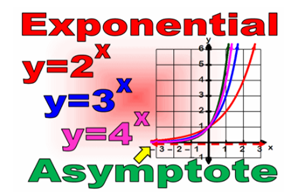117 Lesson 5 - Exponential Function in Excel 01:09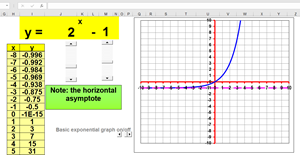118 Lesson 6 - Exponential Formulas (pdf) 00:55

A few exponential equations for your perusal.

119 Lesson 7 - Logarithms: General 09:41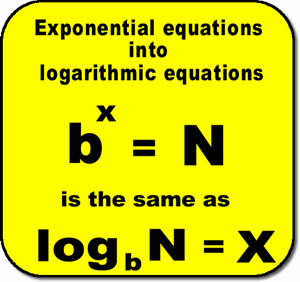120 Lesson 8 - Properties of Logarithmic Functions 08:33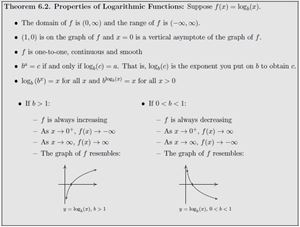121 Lesson 9 - Algebraic Properties of Logarithms 11:48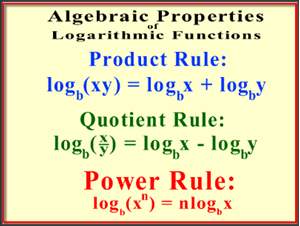122 Lesson 10 - Logarithms: Change of Base Rule 03:23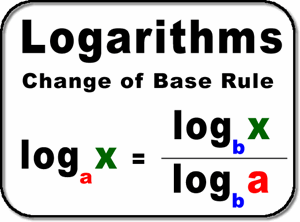123 Lesson 11 - Solving Log Equations 01:59

Solving Log Equations

125 Lesson 12 - Euler's Number 03:33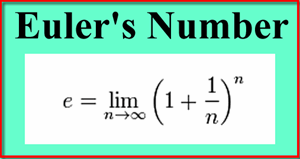126 Lesson 13 - Logarithms - Solving Natural Log Equations 02:01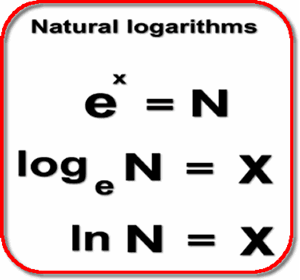127 Lesson 14 - Graphing Logarithmic Functions in Excel 09:46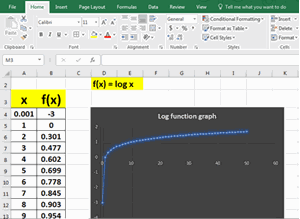128 Lesson 15 - Applications (INTEREST problems only) 10:46

Applications of Exponential and Log Equations (INTEREST problems only)

129 Exponents and Logarithms Worksheet 05:05

Some harder log problems worked out

131 Section 6: Exponents and Logarithms Quiz 00:44

Section 6: Exponents and Logarithms Quiz (Answers under Resources)

132 Section 6 - Brain Candy 05:33

Section 6 - Brain Candy

133 PRACTICE Midterm 3 (pdf) 00:00

PRACTICE Midterm 3 (pdf)

136 Lesson 1 - Systems of Linear Equations 03:27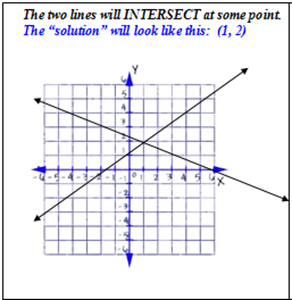137 Lesson 2 - Graphing Systems of Linear Equations 10:47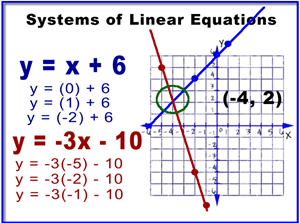139 Lesson 3 - Solving by Substitution 05:09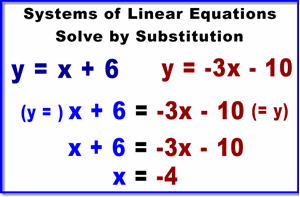141 Lesson 4 - Gaussian Elimination 04:27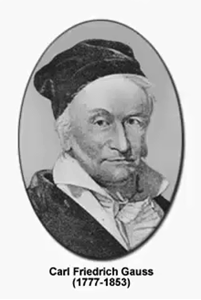143 Lesson 5 - Consistent and Independent Systems 06:15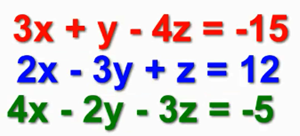144 Lesson 6 - Inconsistent Systems 03:35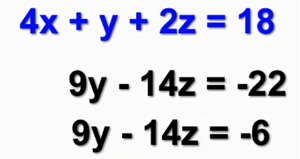145 Lesson 7 - Dependent and Consistent Systems 05:34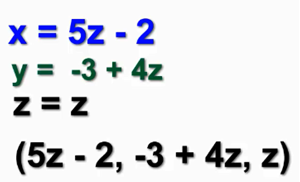147 Lesson 8 - A Coffee Mixture Problem 04:14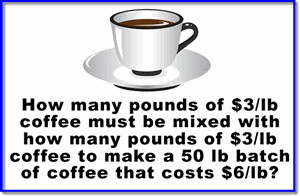148 Lesson 9 - Changing a System into a Matrix 01:59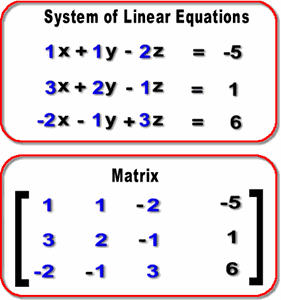149 Lesson 10 - The Matrix - Aij Identifying Rows and Columns 01:10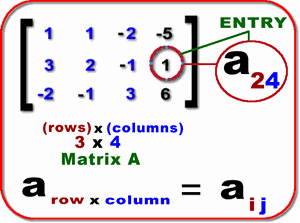150 Lesson 11 - Determinants of a Matrix 06:42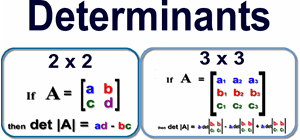151 Lesson 12 - Scalars 01:07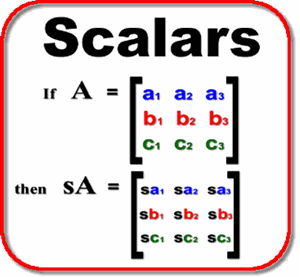152 Determinants and Scalars Worksheet ANSWERS 06:35

Determinants of a Matrix and Scalars Worksheet ANSWERS

153 Lesson 13 - The Augmented Matrix 01:37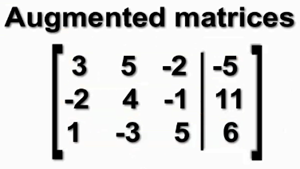154 Lesson 14 - Row Echelon Form 04:17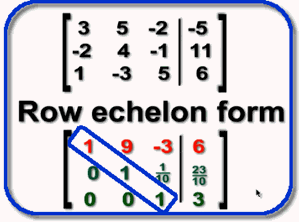155 Lesson 15 - Reduced Row Echelon Form 05:12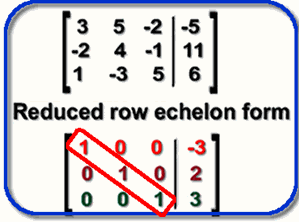156 Lesson 16 - Solving a 3 x 4 matrix 04:34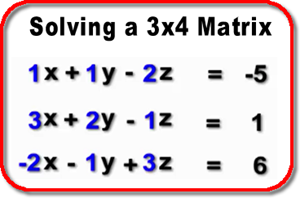158 Lesson 17 - Use a Matrix to Find a Quadratic Equation 06:09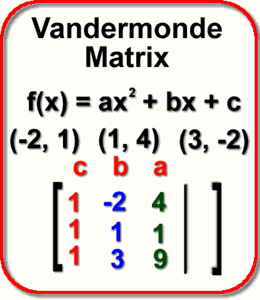160 Lesson 19 - Properties of Matrices 02:48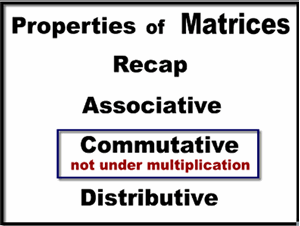161 Lesson 20 - Matrix Addition and Subtraction 04:40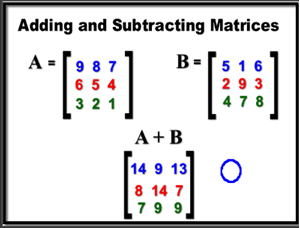162 Lesson 21 - Matrix Multiplication (non-square matrices) 06:44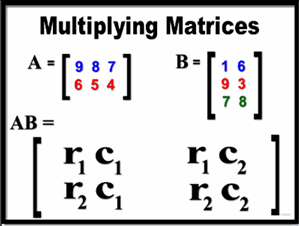163 Lesson 22 - Multiplying Matrices (square matrices) 03:17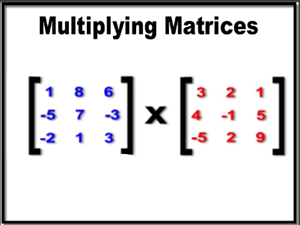165 Lesson 23 - The Identity Matrix 01:44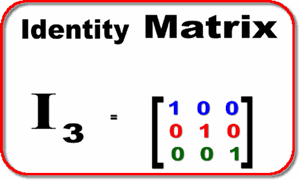166 Lesson 24 - Matrix Inverses 03:42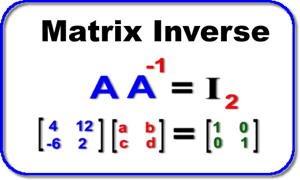168 Lesson 25 - Eigenvalues and Eigenvectors 06:24169 Section 7: Systems of Linear Equations Test (in pdf format) 00:00

Section 7: Systems of Linear Equations Test (in pdf format)  (Answers under Resources)

170 Section 7 - Brain Candy 06:57

Section 7 - Brain Candy

171 Lesson 1 - Sequences 08:23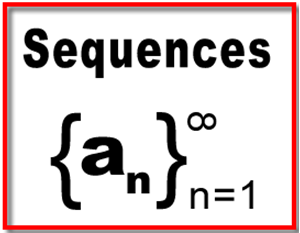172 Lesson 2 - The nth Term 03:40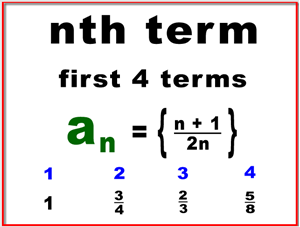173 Lesson 3 - Arithmetic Sequences: Finding the nth Term 02:04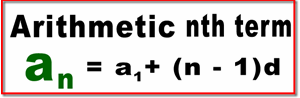174 Lesson 4 - Geometric Sequences: Finding the nth Term 03:49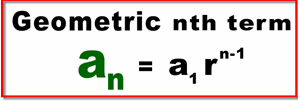176 Lesson 5 - Summation Notation 03:57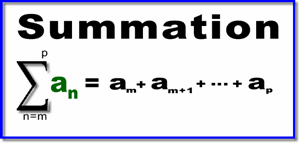177 Lesson 6 - Summation of Arithmetic Sequences 02:10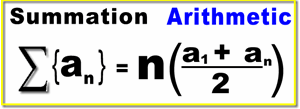178 Lesson 7 - Summation of Geometric Sequences When |r| ≥ 1 02:30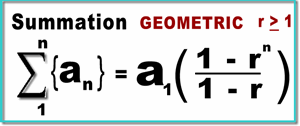179 Lesson 8 - Summation of Geometric Sequences When |r| < 1 03:04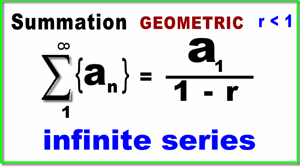181 Lesson 9 - Principal of Mathematical Induction (PMI) 07:54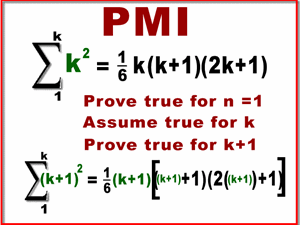183 Lesson 10 - The Binomial Theorem 09:57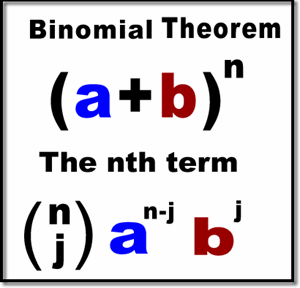185 Section 8: Sequences Test (in pdf format) 00:00

Section 8: Sequences Test (in pdf format) (Answers under Resources)

186 Section 8 - Brain Candy 02:46

Section 8 - Brain Candy

187 PRACTICE Final Exam 00:00

PRACTICE Final Exam

190 Final Exam (pdf) 00:00

Final Exam - cumulative (Answers under Resources)

COURSE LEARNING OUTCOMES

Upon successful completion of this course, students will be able to:

• Write out and describe sets using set-theory notation
• Solve intersections and unions of sets; Venn Diagrams
• Understand the Fundamental Graphing Theory
• Determine the x-intercepts and y-intercepts of polynomial functions
• How to reflect a point and a line across an axis and/or the origin
• Find midpoints and distance between any two given points
• Apply Coordinate Geometry to explore geometric shapes on the Cartesian plane
• Determine if a relation is also a function
• How to graph relations
• How to graph using set-building notation
• Identify the domain and range of a function
• Solve and graph linear equations and inequalities
• Apply step-wise functions
• Solve and graph absolute value equations and inequalities
• Factor polynomials, solve and graph quadratic equations and inequalities
• Calculate with complex numbers, conjugates, discriminants, and real solutions
• Solve quadratic equations by Completing the Square, and with the Quadratic Formula
• Solve application problems including quadratic models and optimization
• Understand and apply the Fundamental Theorem of Algebra
• Determine which functions are polynomial functions and which are not
• Find all of the “zeros” (Real and/or Complex) of a polynomial function
• Define multiplicities of zeros for polynomial functions
• Understand and apply the Factor and Remainder Theorem
• Perform operations on functions, including addition, subtraction, multiplication and division
• Perform synthetic division with polynomial functions
• Understand and apply the Difference Quotient Function
• Find the average rate of change of functions
• Successfully compose functions and define their domains and ranges
• Understand what makes a function one-to-one and/or onto
• Calculate the inverse of a polynomial function, including the domain and range
• Understand and apply the Properties of Exponential Functions
• How to graph exponential functions using MS Excel
• Reduce and calculate with radicals and fractional exponents
• Understand and apply the Properties of Logarithmic Functions
• Apply the rules of logarithms to expand logarithms, or to write logarithmic expressions as a single log
• Solve equations and applications involving exponential and logarithmic functions
• How to graph logarithmic functions using MS Excel
• Calculate with Euler’s number (e)
• To solve logarithmic equations with the Change of Base rule
• Apply exponential and logarithmic properties to real-world problems
• Solve systems of linear equations using the Substitution and/or the Gaussian Elimination method
• Translate systems of linear equations into matrices
• Find determinants of 2 x 2 and 3 x 3 matrices
• To convert any augmented matrix into reduced-row echelon form
• Identify if a matrix is consistent and/or dependent
• Understand what the Identity Matrix is and how it is used
• Perform arithmetic with matrices
• Find inverses of matrices
• Find Eigenvalues and Eigenvectors of 3 x 3 matrices
• To calculate information from a sequence (arithmetic and geometric) such as the sum, nth term and other information
• To use the Principal of Mathematical Induction to prove or disprove statements with Natural numbers
• To apply the Binomial Theorem
• Brain Candy

TARGET AUDIENCE

This is a robust 11th grade algebra course that was designed for high school students planning on attending, and graduating from, college.

COURSE REQUIREMENTS

Students taking this course should ALREADY have basic algebra skills, i.e., arithmetic with fractions, decimals, and percents; arithmetic with integers (a negative multiplied by a negative is a positive); how to algebraically move a variable and/or a constant from one side of an equation to the other side; you know - the absolute basics of algebra. A graphing calculator would be nice but not necessary. A calculator that can calculate exponents and logarithms is needed.

THIS COURSE INCLUDES

•  140+ video tutorials
•  Guided homework worksheets covering every concept (answer sheets with video explanations)
•  8 tests (one for each section)
•  3 Midterms + 3 Practice Midterms (with answers)
•  1 Final Exam + 1 Practice Final Exam (cumulative, with answers)

COURSE CONCEPTS

Basic Algebra Review

• Basic Algebra Review

Introductory Set Theory

• Lesson 1 – Set Theory
• Lesson 2 - Sets of Numbers
• Lesson 3 – Symbols Used in Set Theory
• Lesson 4 - Set Notation
• Lesson 5 - Subsets
• Lesson 6 - Unions and Intersections of Sets
• Lesson 7 - Venn Diagrams

The Cartesian Plane and Coordinate Geometry

• Lesson 1 - The Cartesian Plane
• Lesson 2 - The Fundamental Graphing Principle
• Lesson 3 - Vertical and Horizontal Lines on a Graph
• Lesson 4 - Reflections on a Graph
• Lesson 5 - Intercepts
• Lesson 6 - Midpoints
• Lesson 7 - Distance Formula
• Lesson 8 - Finding points on a graph with a fixed distance
• Lesson 9 - Triangle XYZ is a right triangle
• Lesson 10 - Find the third vertex of a 30-60-90-degree triangle
• Lesson 11 - Find the fourth point of a square
• Lesson 12 - Area of circle and a triangle
• Lesson 13 - Right triangle problem without coordinates

Functions (General) and Graphing

• Lesson 1 - Relations
• Lesson 2 - Graphing Relations using Set Notation
• Lesson 2b - Set notation in a plane
• Lesson 3 - Function notation
• Lesson 4 - Domains and Ranges of Functions
• Lesson 5 - Linear Functions: Slope
• Lesson 6 - Linear Functions Graphing
• Lesson 7 - Linear Equations: Solving
• Lesson 8 - Linear inequalities: Graphing
• Lesson 9 - Linear Functions Worksheet
• Lesson 10 - Step-wise Functions (Piece-wise)
• Lesson 11 - Absolute Value Functions
• Lesson 12 - Absolute Value Inequalities
• Lesson 13 - Absolute Value Inequalities Graphing

• Lesson 1 - Graphing Quadratic Functions
• Lesson 2 - Graphing Quadratic Functions with x intercepts
• Lesson 3 - Imaginary Numbers
• Lesson 4 - Complex Numbers
• Lesson 5 - Graphing Quadratic Equations with no x intercepts
• Lesson 6 - Solving Quadratic Equations by Completing the Square
• Lesson 8 - Graphing Quadratic Inequalities
• Lesson 9 - Minimums and maximums of quadratic functions
• Lesson 10 - Optimization of quadratic functions word problems

Polynomial Functions

• Lesson 1 - Fundamental Theorem of Algebra
• Lesson 2 - Polynomial Function Definition
• Lesson 3 - What is a Polynomial Function?
• Lesson 4 - Function Arithmetic
• Lesson 5 - Finding a “Zero” of a Function
• Lesson 6 - Multiplicities of a Zero
• Lesson 7 - The Factor and The Remainder Theorems
• Lesson 8 - Polynomial Division
• Lesson 9 - Synthetic Polynomial Division
• Lesson 9b - Synthetic Polynomial Division another example
• Lesson 10 - Difference Quotient Function
• Lesson 11 - Average Rate of Change Function
• Lesson 12 - Function Composition
• Lesson 14 - One-to-one and Onto Functions
• Lesson 15 - Inverse Functions

Exponents and Logarithms

• Lesson 1 - Rules of Exponents
• Lesson 2 - Rational Exponents
• Lesson 3 - Exponential Equations
• Lesson 4 - Properties of Exponential Functions
• Lesson 5 - Graphing an Exponential Function in Excel
• Lesson 6 - Exponential Formulas (pdf)
• Lesson 7 - Logarithms: General
• Lesson 8 - Properties of Logarithmic Functions
• Lesson 9 - Algebraic Properties of Logarithms
• Lesson 10 - Solving Logarithmic Equations
• Lesson 11 - Euler's Number
• Lesson 12 - Logarithms - Solving Natural Logarithmic Equations
• Lesson 13 - Graphing Logarithmic Functions in Excel
• Lesson 14 - Applications (INTEREST problems only)
• Lesson 15 - Logarithms: Change of Base Rule
• Lesson 16 - Some Exponential and Logarithmic Problems Worked Out

Systems of Equations

• Lesson 1 - Systems of Linear Equations
• Lesson 2 - Graphing Systems of Linear Equations
• Lesson 3 - Solving by Substitution
• Lesson 4 - Gaussian Elimination
• Lesson 5 - Consistent and Independent Systems (3 variables)
• Lesson 6 - Inconsistent Systems (3 variables)
• Lesson 7 - Dependent and Consistent Systems (3 variables)
• Lesson 8 - Coffee mixture problem
• Lesson 9 - Changing a System into a Matrix
• Lesson 10 - The Matrix - Aij Identifying Rows and Column
• Lesson 11 - Determinants of a Matrix
• Lesson 12 - The Augmented Matrix
• Lesson 13 - Row Echelon Form
• Lesson 14 - Reduced Row Echelon Form
• Lesson 15 - Solving a 3 x 4 matrix
• Lesson 16 - Matrix - Inconsistent and/or Dependent
• Lesson 17 - How to Use a Matrix to Find a Quadratic Equation
• Lesson 18 - Scalars
• Lesson 19 - Properties of Matrices
• Lesson 20 - Matrix Addition and Subtraction
• Lesson 21 - Matrix Multiplication (non-square matrices)
• Lesson 22 - Multiplying Matrices (square matrices)
• Lesson 23 - The Identity Matrix
• Lesson 24 - Matrix Inverses
• Lesson 25 - Eigenvalues and Eigenvectors

Sequences

• Lesson 1 - Sequences
• Lesson 2 - The nth Term
• Lesson 3 - Arithmetic Sequences: Finding the nth Term
• Lesson 4 - Geometric Sequences: Finding the nth Term
• Lesson 5 - Summation Notation
• Lesson 6 - Summation of Arithmetic Sequences
• Lesson 7 - Summation of Geometric Sequences When |r| ≥ 1
• Lesson 8 - Summation of Geometric Sequences When |r| < 1
• Lesson 9 - Principal of Mathematical Induction (PMI)
• Lesson 10 - The Binomial Theorem
• Teacher: Richard
• Areas of expertise: Algebra, Statistics and Probability, Trigonometry, Calculus, Discrete Math, Linear Algebra, How to Teach Math (for future Math instructors), Business Math, and others.
• Education: BA in Mathematics (SDSU), MA in Curriculum and Instruction Emphasis in Math Instruction (SDSU), BCLAD Teaching Credential (SDSU)
• Interests: Watching students succeed in mathematics, Emerging Learning Technologies, Puzzles, Soduko, Space Exploration, Science Fiction, Zombie movies (good ones, not those cheap ones!), Music, Video Production, Website Construction, World Peace, Family, others
• Skills: I have an easy non-threatening approach to the teaching/learning of mathematics. I break down the harder concepts into easier to understand steps. I am one of those weirdos who actually loves math. I see math like art and/or music - it gives me goose bumps!
• Associations: TODOS: Mathematics For All Greater San Diego Math Teachers Association National Council of Math Teachers (NCTM) Hispanic Association of Colleges and Universities (HACU) White House Initiative on Educational Excellence for Hispanic Americans’ Hispanic Family Learning Initiative
• Issues I care about: I am very concerned with how our country has watered down math education in our public schools. There is no doubt that the courses that keeps students from succeeding in high school and/or college are all mathematics courses. I don't blame the students - I blame the educators; not the teachers, but the Educational Leaders who make the big decisions. Public school teachers have zero choice in what and how to teach math.

I have been a Math Learning Specialist and Director of our University's Tutoring Center for the last 15 years. I have personally worked with thousands of struggling math students. I have also taught all of the math courses that our school offers, at both undergraduate and graduate level. A very sad fact is that around 90% of our incoming students are not prepared for college level mathematics. For some strange reason, students who are not successful at math in high school believe that they will understand it when they get to college - just the opposite is true. Students who master the concepts covered in this 11th grade course will succeed in their math courses in college.

What you should know before beginning this course

What you should already know before beginning this course

Section 1: Set Theory Quiz ANSWERS

Section 1: Set Theory Quiz ANSWERS

Section 2: Cartesian Plane and Coordinate Geometry Quiz ANSWERS

Section 2: Cartesian Plane and Coordinate Geometry Quiz ANSWERS

Section 3: Functions (General) and Graphing Quiz ANSWERS

Section 3: Functions (General) and Graphing Quiz ANSWERS

Section 5: Polynomial Functions Quiz ANSWERS

Section 5: Polynomial Functions Quiz ANSWERS

Section 6: Exponents and Logarithms Quiz ANSWERS

Section 6: Exponents and Logarithms Quiz ANSWERS

Section 7: Systems of Linear Equations Test ANSWERS

Section 7: Systems of Linear Equations Test ANSWERS

Graphing Linear Equations (pdf)

Graphing Linear Equations (pdf)

Graphing blanks for practice

Graphing blanks for practice

Basic Geometry Formulas

Basic Geometry Formulas used in this course.

List of Powers 2 - 20

List of Powers 2 - 20 - KNOW THEM!

Systems of Linear Equations: Example

Systems of Linear Equations: Example
Customers who bought this item also boughtThis High School elective course can be used as a Science or Career and Technical Education(CTE) course. Topics covered include: Video game history, careers, design basics, developing/presenting your game concept, creating games with the Unity game engine, C# programming, and more. +
\$349.00Explore the great literature of the world from the time of Homer to the present. This is a Grade 11 and 12 English course that will encompass most of the Common Core standards, and touch on most of the recommended Common Core texts, while also extending beyond that to survey world literature. +
\$149.00This engaging course covers all the topics for the full 7th Grade Math year. We will cover: (1) Proportional Reasoning (2) Rational Numbers (3) Expressions and Equations (4) Geometry (5) Statistics & Probability +
\$299.00 \$129.00